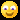# Gravity & Magnetic Surveying

20 Questions | Attempts: 3258SettingsThis Quiz contains the questions from the basics and applications of Gravity and Magnetic methods used in Geophysical Investigations. Enjoy the Quiz n Tk cr. . . . . Be Happy. . . All the best. . . . .

• 1.
Gravity field variations on the surface :
• A.

Reflects the density variation laterally in the subsurface

• B.

Reflects the density variation vertically in the subsurface

• C.

Do not reflect density variation

• D.

Reflect topogrphic variations only

• 2.
Sensitivity of a Gravimeter is :
• A.

0.01 mgal

• B.

0.1 mgal

• C.

0.002 mgal

• D.

0.001 mgal

• 3.
Optical Pumping magnetometer works on the pinciple of:
• A.

Doppler effect

• B.

Proton Precession

• C.

Magnetic saturation

• D.

Zeeman effect

• 4.
The half width of vertical magnetic intensity anomaly over a magnetised sphere is 25 meters .  Calculate the depth to the center of sphere from surface ??
• A.

32.5 m

• B.

50 m

• C.

25 m

• D.

None of these

• 5.
Van allen zones form at :
• A.

North Pole

• B.

South Pole

• C.

North and South both

• D.

Equator

• 6.
The scale of Geodetic Survey is :
• A.

1:250000

• B.

1:10000

• C.

1:5000

• D.

1:50000

• 7.
At magnetic equator, the inclination of total magnetic field is :
• A.

90 degree

• B.

60 degree

• C.

45 degree

• D.

0 degree

• 8.
A gravity profile across a schist belt within a Granitic country rock will give rise to a :
• A.

Central low

• B.

Central High

• C.

Response is flat

• D.

High and Low at the edges

• 9.
Which of the following is non-magnetic ???
• A.

Granite

• B.

Gabbro

• C.

Limestone

• D.

Diorite

• 10.
A large sedimenary basin 2 km deep with density contrast of 0.3 gm/cc will approximately give rise to gravity anomaly of :
• A.

5 mgal

• B.

15 mgal

• C.

25 mgal

• D.

50 mgal

• 11.
Relationship of geometrical and gravitational flattening of Earth is given by :
• A.

Gauss Theorem

• B.

Green's Theorem

• C.

Hayford's Theorem

• D.

Clairaut's Theorem

• 12.
The Bouguer anomaly over an isostatically compensated region is :
• A.

Zero

• B.

Positive

• C.

Negative

• D.

Same as isostatic anomaly

• 13.
Magnetic equator coincides with the Geomagnetic equator.
• A.

True

• B.

False

• 14.
SI unit of manetic induction is
• A.

Tesla

• B.

Gauss

• C.

Oesterd

• D.

None of above

• 15.
The magnetic domains are due to :
• A.

Covalent bonds

• B.

Doping

• C.

Hysteresis loss

• D.

Spin excange interaction

• 16.
Terrain correction is not generally applied to magnetic field data .
• A.

True

• B.

False

• 17.
Curie point temperature for Haematite is :
• A.

578 degree celsius

• B.

670 degree celsius

• C.

550 degree celsius

• D.

None of these

• 18.
Which of the following exhibits negative magnetic susceptibility ???
• A.

Pyroxene

• B.

Quartz

• C.

Olivine

• D.

Biotite

• 19.
To derive magnetic field from gravity field , the poisson relation can be used only when the direction of magnetization is :
• A.

Horizontal ( 0 degree)

• B.

45 degree

• C.

60 degree

• D.

Vertical ( 90 degree)

• 20.
Gravity measurement is made on a ship sailing at the speed of 6 knots in the direction N650E at 200N latitude. The Eotvos correction (in mGal) is :
• A.

+ 38.5

• B.

+ 24.5

• C.

- 35.5

• D.

-39.5

## Related TopicsBack to top
×

Wait!
Here's an interesting quiz for you.